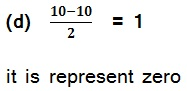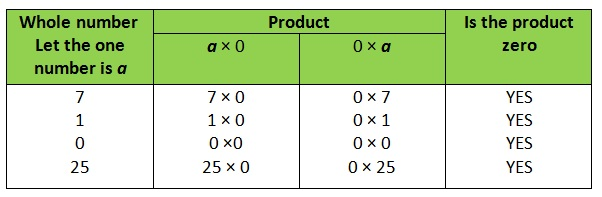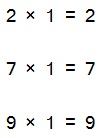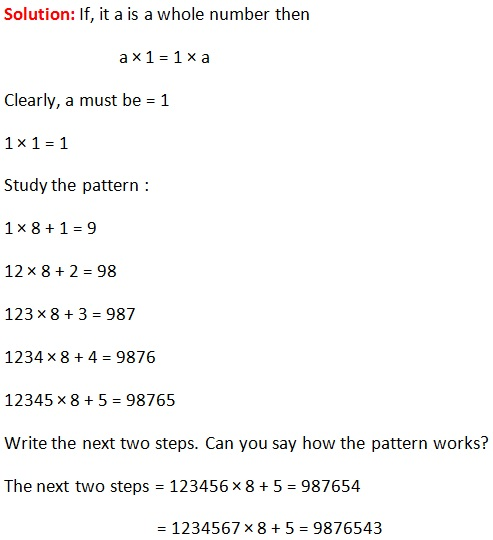Study Materials: ncert solutions

Our ncert solutions for Exercise 2.3 Class 6 maths 2. Whole Numbers - ncert solutions - Toppers Study is the best material for English Medium students cbse board and other state boards students.

Solutions ⇒ Class 6th ⇒ Mathematics ⇒ 2. Whole Numbers

# Exercise 2.3 Class 6 maths 2. Whole Numbers - ncert solutions - Toppers Study

Topper Study classes prepares ncert solutions on practical base problems and comes out with the best result that helps the students and teachers as well as tutors and so many ecademic coaching classes that they need in practical life. Our ncert solutions for Exercise 2.3 Class 6 maths 2. Whole Numbers - ncert solutions - Toppers Study is the best material for English Medium students cbse board and other state boards students.

## Exercise 2.3 Class 6 maths 2. Whole Numbers - ncert solutions - Toppers Study

CBSE board students who preparing for class 6 ncert solutions maths and Mathematics solved exercise chapter 2. Whole Numbers available and this helps in upcoming exams 2023-2024.

### You can Find Mathematics solution Class 6 Chapter 2. Whole Numbers

• All Chapter review quick revision notes for chapter 2. Whole Numbers Class 6
• NCERT Solutions And Textual questions Answers Class 6 Mathematics
• Extra NCERT Book questions Answers Class 6 Mathematics
• Importatnt key points with additional Assignment and questions bank solved.

NCERT Solutions do not only help you to cover your syllabus but also will give to textual support in exams 2023-2024 to complete exercise 2.3 maths class 6 chapter 2 in english medium. So revise and practice these all cbse study materials like class 6 maths chapter 2.3 in english ncert book. Also ensure to repractice all syllabus within time or before board exams for ncert class 6 maths ex 2.3 in english.

See all solutions for class 6 maths chapter 2 exercise 2 in english medium solved questions with answers.

### Exercise 2.3 class 6 Mathematics Chapter 2. Whole Numbers

• Exercise 2.3 Class 6 Maths 2. Whole Numbers - Ncert Solutions - Toppers Study
• Class 6 Ncert Solutions
• Solution Chapter 2. Whole Numbers Class 6
• Solutions Class 6
• Chapter 2. Whole Numbers Exercise 2.3 Class 6

## Exercise 2.3 Class 6 maths 2. Whole Numbers - ncert solutions - Toppers Study

### Exercise-2.3

Q1. Which of the following will not represent zero:
(a) 1 + 0 (b) 0 × 0 (c) 0/2     (d) 10 -10/

Solution:

(a) 1 + 0  = 1

So, it is not represent zero

(b) 0 × 0 = 0

it is represent zero

(c)  0/2 = 0

it is represent zeroQ2. If the product of two whole numbers is zero, can we say that one or both of them will be zero? Justify through examples.

Solution:

Yes, we know that the product of any whole numbers with zero is always zero.Q3. If the product of two whole numbers is 1, can we say that one or both of them will be 1? Justify through examples.

Solution:

If only one number be 1 then the product cannot be 1.

Examples:Here we see that one of two is 1 then product will be always second number.

If both numbers are 1, then the product is 1

Q4. Find using distributive property :
(a) 728 × 101    (b) 5437 × 1001    (c) 824 × 25    (d) 4275 × 125 (e) 504 × 35

Solution:

(a) 728 × 101

= 728(100 + 1)

= 728 × 100 + 728 × 1

= 72800 + 728

= 73528

Solution:

(b) 5437 × 1001

= 5437(1000 + 1)

= 5437 × 1000 + 5437 × 1

= 5437000 + 5437

= 5442437

Solution:

(c) 824 × 25

= 824(20 + 5)

= 824 × 20 + 824 × 5

= 16480 + 4120

20600

Solution:

(d) 4275 × 125

= 4275(100 + 20 + 5)

= 4275 × 100 + 4275 × 20 + 4275 × 5

= 427500 + 85500 + 21375

= 534375

Solution:

(e) 504 × 35

= (500 + 4) × 35

= 500 × 35 + 4 × 35

= 17500 + 140

= 17640

Q5. Study the pattern :
1 × 8 + 1 = 9       1234 × 8 + 4 = 9876
12 × 8 + 2 = 98     12345 × 8 + 5 = 98765
123 × 8 + 3 = 987
Write the next two steps. Can you say how the pattern works?
(Hint: 12345 = 11111 + 1111 + 111 + 11 + 1).##### Other Pages of this Chapter: 2. Whole Numbers

Important Study materials for classes 06, 07, 08,09,10, 11 and 12. Like CBSE Notes, Notes for Science, Notes for maths, Notes for Social Science, Notes for Accountancy, Notes for Economics, Notes for political Science, Noes for History, Notes For Bussiness Study, Physical Educations, Sample Papers, Test Papers, Mock Test Papers, Support Materials and Books.Mathematics Class - 11th

NCERT Maths book for CBSE Students.

books

## Study Materials List:

##### Solutions ⇒ Class 6th ⇒ Mathematics
1. Knowing Our Numbers
2. Whole Numbers
3. Playing with Numbers
4. Basic Geometrical Ideas
5. Understanding Elementary Shapes
6. Integers
7. Fractions
8. Decimals

New Books